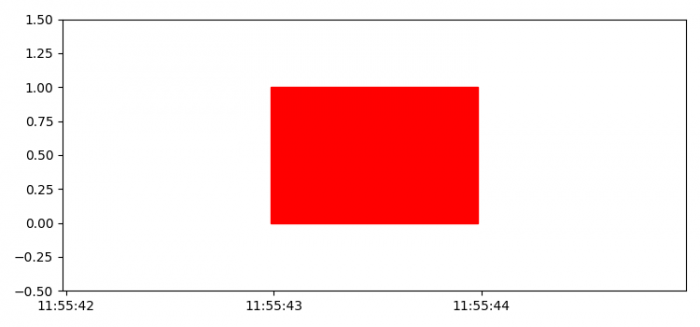# How to plot a rectangle on a datetime axis using Matplotlib?

To plot a recatangle on a datetime axis using matplotlib, we can take the following steps −

• Set the figure size and adjust the padding between and around the subplots.
• Create a new figure or activate an existing figure.
• Add an '~.axes.Axes' to the figure as part of a subplot arrangement using add_subplot() method.
• To define a rectangle, find the anchor points using datetime and matplotlib's dates.
• Set major axis locator and formatter.
• Limit x and y axes scale.
• To display the figure, use show() method.

## Example

from datetime import datetime, timedelta
from matplotlib.patches import Rectangle

import matplotlib.pyplot as plt
import matplotlib.dates as mdates

plt.rcParams["figure.figsize"] = [7.50, 3.50]
plt.rcParams["figure.autolayout"] = True

fig = plt.figure()

startTime = datetime.now()
endTime = startTime + timedelta(seconds=1)
start = mdates.date2num(startTime)
end = mdates.date2num(endTime)

width = end – start
rect = Rectangle((start, 0), width, 1, color='red')

locator = mdates.AutoDateLocator(minticks=3)
formatter = mdates.AutoDateFormatter(locator)

ax.xaxis.set_major_locator(locator)
ax.xaxis.set_major_formatter(formatter)

plt.xlim([start - width, end + width])
plt.ylim([-.5, 1.5])

plt.show()

## Output